# 投射余可解的Gorenstein平坦模和Gorenstein AC投射模Projectively Coresolved Gorenstein Flat Modules and Gorenstein AC Projective Modules

DOI: 10.12677/PM.2021.114074, PDF, HTML, XML, 下载: 17  浏览: 90  国家自然科学基金支持

Abstract: In this paper, we give a condition in order for the class of Gorenstein AC projective modules to co-incide with the class of projectively coresolved Gorenstein flat modules, we prove that the class of Gorenstein AC projective modules equal to the class of projectively coresolved Gorenstein flat modules equal to the class of Ding projective modules over coherent rings. We also give a necessary and sufficient condition in order for the class of Gorenstein AC projective modules to coincide with the class of Gorenstein projective modules, we prove that the class of Gorenstein AC projective modules to coincide with the class of Gorenstein projective modules if and only if the class of level modules belongs to the right orthogonal class with respect to Gorenstein projective modules. And we give some necessary and sufficient conditions in order for the class of Gorenstein AC projective modules to coincide with the class of Gorenstein projective modules over coherent rings.

1. 引言

2. 预备知识

${\mathcal{X}}^{\perp }=\left\{Y\in M\left(R\right)|{\text{Ext}}_{R}^{1}\left(X,Y\right)=0,任意的\text{ }X\in \mathcal{X}\right\}$

${}^{\perp }\mathcal{X}=\left\{Y\in M\left(R\right)|{\text{Ext}}_{R}^{1}\left(Y,X\right)=0,任意的\text{ }X\in \mathcal{X}\right\}$

$\cdots \to {P}_{2}\to {P}_{1}\to {P}_{0}\to F\to 0$

2) 称R-模L是Level模，如果对任意的超有限表示模F，有 ${\text{Tor}}_{1}^{R}\left(F,L\right)=0$

3) 称R-模A是绝对clean模，如果对任意的超有限表示模F，有 ${\text{Ext}}_{R}^{1}\left(F,A\right)=0$

$ℙ:\cdots \to {P}_{1}\to {P}_{0}\to {P}^{0}\to {P}^{1}\to \cdots$

1) 若对任意的投射R-模Q，有 ${\text{Hom}}_{R}\left(ℙ,Q\right)$ 正合，则称R-模M是Gorenstein投射模。用 $\mathcal{G}\mathcal{P}$ 表示Gorenstein投射模的类。

2) 若对任意的平坦R-模F，有 ${\text{Hom}}_{R}\left(ℙ,F\right)$ 正合，则称R-模M是Ding投射模。用 $\mathcal{D}\mathcal{P}$ 表示Ding投射模的类。

3) 若对任意的Level R-模L，有 ${\text{Hom}}_{R}\left(ℙ,L\right)$ 正合，则称R-模M是Gorenstein AC投射模。用 $\mathcal{G}{\mathcal{P}}_{\text{ac}}$ 表示Gorenstein AC投射模的类。

4) 若对任意的内射右R-模I，有 $I{\otimes }_{R}ℙ$ 正合，则称R-模M是投射余可解的Gorenstein平坦模。用 $\mathcal{P}\mathcal{G}\mathcal{F}$ 表示投射余可解的Gorenstein平坦模的类。

$\mathbb{F}:\cdots \to {F}_{1}\to {F}_{0}\to {F}^{0}\to {F}^{1}\to \cdots$

$ℙ:\cdots \to P\to P\to P\to P\to \cdots$

3. 主要结果

1) $\mathcal{G}{\mathcal{P}}_{\text{ac}}\subseteq \mathcal{G}\mathcal{F}$

2) 对任意的R-模 $M\in \mathcal{G}{\mathcal{P}}_{\text{ac}}$，有 ${M}^{+}\in \mathcal{G}{\mathcal{I}}_{\text{}}$

3) ${\mathcal{I}}^{+}\subseteq \mathcal{G}{\mathcal{P}}_{\text{ac}}^{\perp }$

2) ⟹ 1) 设 $M\in \mathcal{G}{\mathcal{P}}_{\text{ac}}$。则存在投射R-模的正合列

$ℙ:\cdots \to {P}_{1}\to {P}_{0}\to {P}_{-1}\to {P}_{-2}\to \cdots$

${ℙ}^{+}:\cdots \to {P}_{-1}^{+}\to {P}_{0}^{+}\to {P}_{1}^{+}\to {P}_{2}^{+}\to \cdots$

2) ⟹ 3) 设 $M\in \mathcal{G}{\mathcal{P}}_{\text{ac}}$。则 ${M}^{+}\in \mathcal{G}\mathcal{I}$。从而对任意的内射右R-模I，有

${\text{Ext}}_{R}^{1}\left(M,{I}^{+}\right)\cong {\text{Ext}}_{R}^{1}\left(I,{M}^{+}\right)=0$

3) ⟹ 2) 设 $M\in \mathcal{G}{\mathcal{P}}_{\text{ac}}$。则存在投射R-模的正合列

$ℙ:\cdots \to {P}_{1}\to {P}_{0}\to {P}_{-1}\to {P}_{-2}\to \cdots$

$ℙ:\cdots \to {P}_{1}\to {P}_{0}\to {P}^{0}\to {P}^{1}\to \cdots$

$ℙ:\cdots \to {P}_{1}\to {P}_{0}\to {P}^{0}\to {P}^{1}\to \cdots$

$ℙ:\cdots \to {P}_{1}\to {P}_{0}\to {P}_{-1}\to {P}_{-2}\to \cdots$

1) $\mathcal{G}\mathcal{P}\subseteq \mathcal{G}\mathcal{F}$

2) $\mathcal{G}{\mathcal{P}}_{\text{ac}}=\mathcal{G}\mathcal{P}$

3) 对任意的R-模 $M\in \mathcal{G}\mathcal{P}$，有 ${M}^{+}\in \mathcal{G}\mathcal{I}$

4) ${\mathcal{I}}^{+}\subseteq \mathcal{G}{\mathcal{P}}^{\perp }$

1) ⟹ 2) 由定义知，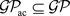。下证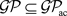。设M是强Gorenstein投射模。则存在投射R-模的正合列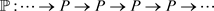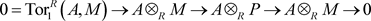1) ⇔ 3) 由( ，定理2.2)可得。

3) ⟹ 4) 设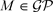。因为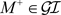，所以对任意的内射右R-模I，有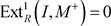。又因为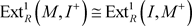，所以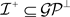4) ⟹ 3) 设。则存在投射R-模的正合列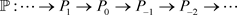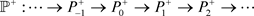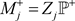。因为，所以对任意的内射右R-模I，有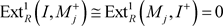。因此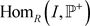正合。故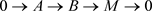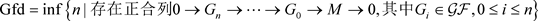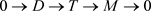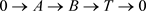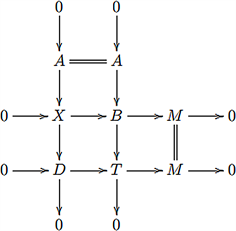Enochs, E.E. and Jenda, O.M.G. (1995) Gorenstein Injective and Gorenstein Projective Modules. Mathematische Zeitschrift, 220, 611-633. https://doi.org/10.1007/BF02572634  Enochs, E.E., Jenda, O.M.G. and Torrecillas, B. (1993) Gorenstein Flat Modules. Annals of Mathematics, Nanjing University, 10, 1-9.  Ding, N., Li, Y. and Mao, L. (2009) Strongly Gorenstein Flat Modules. Journal of the Australian Mathematical Society, 86, 323-338. https://doi.org/10.1017/S1446788708000761  Gillespie, J. (2010) Model Structures on Modules over Ding-Chen Rings. Homology, Homotopy and Applications, 12, 61-73. https://doi.org/10.4310/HHA.2010.v12.n1.a6  Gillespie, J. (2015) On Ding Injective, Ding Projective, and Ding Flat Modules and Complexes. Rocky Mountain Journal of Mathematics, 47, 2641-2673.  Saroch, J. and Stovícek, J. (2020) Singular Compactness and Definability for -Cotorsion and Gorenstein Modules. Selecta Mathematica, 26, 23-63. https://doi.org/10.1007/s00029-020-0543-2  Iacob, A. (2020) Projectively Coresolved Gorenstein Flat and Ding Projective Modules. Communications in Algebra, 48, 2883-2893. https://doi.org/10.1080/00927872.2020.1723612  Bravo, D., Gillespie, J. and Hovey, M. (2014) The Stable Module Category of a General Ring. Mathematics, arxiv: 1210.0196.  Holm, H. (2004) Gorenstein Homological Dimensions. Journal of Pure and Applied Algebra, 189, 167-193. https://doi.org/10.1016/j.jpaa.2003.11.007  Gillespie, J. (2018) Gorenstein AC-Projective Complexes. Journal of Homotopy and Related Structures, 13, 769-791. https://doi.org/10.1007/s40062-018-0203-9  Emmanouil, I. (2012) On the Finiteness of Gorenstein Homo-logical Dimensions. Journal of Algebra, 372, 376-396. https://doi.org/10.1016/j.jalgebra.2012.09.018  Estrada, S., Iacob, A. and Pérez, M. (2017) Model Structures and Relative Gorenstein Flat Modules and Chain Complexes. Contemporary Mathematics, arxiv: 1709.00658.